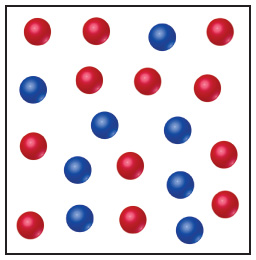# Problem: The following diagram is a representation of 20 atoms of a fictitious element, which we will call nevadium (Nv). The red spheres are 293Nv, and the blue spheres are 295Nv.Assuming that this sample is a statistically representative sample of the element, calculate the percent abundance of each element.

###### FREE Expert Solution

We have the following percent abundance for each of the isotopes.

84% (226 ratings)###### Problem Details

The following diagramis a representation of 20 atoms of a fictitious element, which we will call nevadium (Nv). The red spheres are 293Nv, and the blue spheres are 295Nv.

Assuming that this sample is a statistically representative sample of the element, calculate the percent abundance of each element.

Frequently Asked Questions

What scientific concept do you need to know in order to solve this problem?

Our tutors have indicated that to solve this problem you will need to apply the Atomic Mass concept. You can view video lessons to learn Atomic Mass. Or if you need more Atomic Mass practice, you can also practice Atomic Mass practice problems.

What professor is this problem relevant for?

Based on our data, we think this problem is relevant for Professor Kizilkaya's class at LSU.